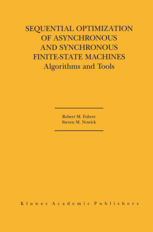## Wednesday, September 12, 2012

### Basic Sequencial Machine Theory and Synchronous Finite State Machines PPT SLIDESBasic Sequencial Machine Theory and Synchronous Finite State Machines
Instructor:Marek A.Perkowski
Textbook: Sequencial Machine Theory and Synchronous Finite State Machines
Download Slides from here

Lecture 1. Introduction to Sequential Machine Theory. Why Sequential Theory, software/hardware. Lecture 2. Equivalence. Deterministic and Non-Deterministic Finite Automata.

Lecture 3. Regular Languages. Languages and Formal Languages. Strings and substrings.

Lecture 4. Deterministic FA/PDA. Numerical Acceptor. Languages accepted by FA.

Lecture 6.  Regular Expression Manipulation FSM model. Null Machine

Lecture 7. Behavioral Equivalence. Examples. Verifying behavioral equivalence of FSMs.

Lecture 8. Morphisms of State Machines. Free semi-groups. Concatenation.

Advanced FSM minimization. In PDF format.

Rule Based State Assignment. In PDF format. Flip-flops. FSM equations.

State assignment using multiline and partitions. In PDF format.

Example of using state assignment based on rules. In PDF format.